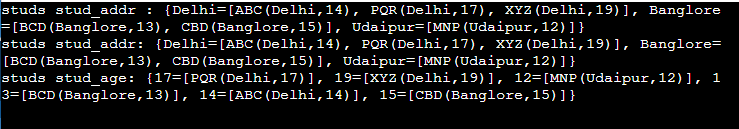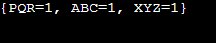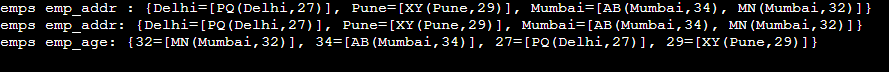# Java 8 Group By

## Introduction to Java 8 Group By

Java 8 group by method permits java developers to perform the operation of group by directly. As we know, group by is a SQL aggregation operation that can be used to order data. It will allow us to categorize the data based on predefined criteria or conditions. In Java, we use the groupingBy method, which was provided by the collector and stream. This method is useful for concurrent collectors.

### Key Takeaways

• In Java, we use the groupingBy method to perform the operation, such as group by method used in SQL.
• We are using a grouping object for the specified property to store the results in a map instance. We use the same method as SQL.

### Overview of Java 8 Group By

In the collector’s class, we use the group by method to group objects by property and store the results in the map instance. In order to perform group by operations in Java, we must specify the property from which we want to perform the group by operations. This Java method performs the same functions as the SQL group by clause. A stream represents the sequence of elements, and it will support various operations that lead to the desired result.

The stream source is a collection of arrays from which the data is streamed. In Java, a stream differs from a collection in multiple ways. The collector class in Java is very versatile and vast, containing multiple methods. We are using the collector groupingBy method to group objects based on a specific property, and the results are stored in a map.

### How to Use Group By in Java 8?

Java 8 allows the group by using the collectos.groupingby method. It is a very useful operation of aggregation.

In the below example, we are creating the class name as grpby; also, in the same class, we are creating the class as stud.

Code:

``````import java.io.IOException;
import java.util.ArrayList;
import java.util.HashMap;
import java.util.List;
import java.util.Map;
import java.util.Objects;
import java.util.stream.Collectors;
public class grpby

public static void main(String[] args) throws IOException

List<stud> st = new ArrayList<> ();
st.add (new stud ("ABC", "Delhi", 14));
st.add (new stud ("PQR", "Delhi", 17));
st.add (new stud ("XYZ", "Delhi", 19));
st.add (new stud ("MNP", "Udaipur", 12));
st.add (new stud ("BCD", "Banglore", 13));
st.add (new stud ("CBD", "Banglore", 15));
for (stud p : st)

Map<Integer,List<stud>> stBystud_age = st.stream().collect(Collectors.groupingBy(stud::getstud_age));
System.out.println ("studs stud_age: " + stBystud_age);

class stud

private String stud_name;
private int stud_age;
public stud (String stud_name, String stud_addr, int stud_age)

this.stud_name = stud_name;
this.stud_age = stud_age;

public String getstud_name ()
return stud_name;

public void setstud_name (String stud_name)
this.stud_name = stud_name;

public int getstud_age ()
return stud_age;

public void setstud_age (int stud_age)
this.stud_age = stud_age;

@Override
public String toString ()
return String.format ("%s(%s,%d)", stud_name, stud_addr, stud_age);

@Override
public int hashCode ()
int hash = 7;
hash = 79 * hash + Objects.hashCode (this.stud_name);
hash = 79 * hash + Objects.hashCode (this.stud_addr);
hash = 79 * hash + this.stud_age;
return hash;

@Override
public boolean equals (Object obj)
if (obj == null)
return false;

if (getClass () != obj.getClass())
return false;

final stud other = (stud) obj;
if (!Objects.equals (this.stud_name, other.stud_name))
return false;

return false;

if (this.stud_age != other.stud_age)
return false;

return true;

``````

Output:### Java 8 Collectors groupingBy() Method

The groupingBy method is used to group the object of specified property and used to store the map instance results.

Below is the syntax of collectors grouping by the method as follows:

Syntax:

``public static collector <T(input element), ?, <k list (type of elements)>>groupingBy (classifier function)``

Below is the parameter description of the java 8 collectors groupingBy method.

This method takes two types of parameters.

• T: This parameter defines the input element type that we are giving with the collectors groupingBy method.
• K: This parameter defines the input elements converted type which we are giving with the collectors groupingBy method.

The java 8 collector’s groupingBy method accepts the two types of parameters.

Below is the parameter of the collector’s groupingBy method as follows:

• Function: This parameter specifies the property that will be applied to the input elements.
• Classifier: This parameter is used to map the input elements from the specified destination map.

The java 8 collectors groupingBy method returns the collectors as a map. The below example shows collectors grouping by method in java 8 as follows:

Code:

``````import java.util.*;
import java.util.function.Function;
import java.util.stream.Collectors;
public class coll_grpby

public static void main(String[] args)

List<String> g
= Arrays.asList ("ABC", "XYZ", "PQR");
Map<String, Long> res
= g.stream ().collect (
Collectors.groupingBy (
Function.identity (),
Collectors.counting ()));
System.out.println (res);

``````

Output:### Example of Java 8 Group By

In the below example, we are creating the class name as grpby. Also, we are defining the new class name as emp.

Code:

``````import java.io.IOException;
import java.util.ArrayList;
import java.util.HashMap;
import java.util.List;
import java.util.Map;
import java.util.Objects;
import java.util.stream.Collectors;
public class grpby

public static void main(String[] args) throws IOException

List<emp> ep = new ArrayList<> ();
for(emp p : ep)

Map<Integer,List<emp>> stByemp_age = ep.stream().collect(Collectors.groupingBy(emp::getemp_age));
System.out.println ("emps emp_age: " + stByemp_age);

class emp

private String emp_name;
private int emp_age;
public emp (String emp_name, String emp_addr, int emp_age)

this.emp_name = emp_name;
this.emp_age = emp_age;

public String getemp_name ()
return emp_name;

public void setemp_name (String emp_name)
this.emp_name = emp_name;

public int getemp_age()
return emp_age;

public void setemp_age (int emp_age)
this.emp_age = emp_age;

@Override
public String toString()

@Override
public int hashCode()
int hash = 7;
hash = 79 * hash + Objects.hashCode(this.emp_name);
hash = 79 * hash + Objects.hashCode(this.emp_addr);
hash = 79 * hash + this.emp_age;
return hash;

@Override
public boolean equals(Object obj)

if (obj == null)

return false;

if (getClass() != obj.getClass())

return false;

final emp other = (emp) obj;
if (!Objects.equals(this.emp_name, other.emp_name))
return false;

return false;

if (this.emp_age != other.emp_age)
return false;

return true;

``````

Output:### Conclusion

We are using the grouping by method in the collectors class to group objects by property and store the results in the map instance. Java 8 group by method allows java developers to perform group by operations directly. As we all know, group by is a SQL aggregation operation that can be used to sort data.

### Recommended Articles

This is a guide to Java 8 Group By. Here we discuss the introduction, how to use group by in java 8, method, and example. You may also have a look at the following articles to learn more –

The post Java 8 Group By appeared first on EDUCBA.